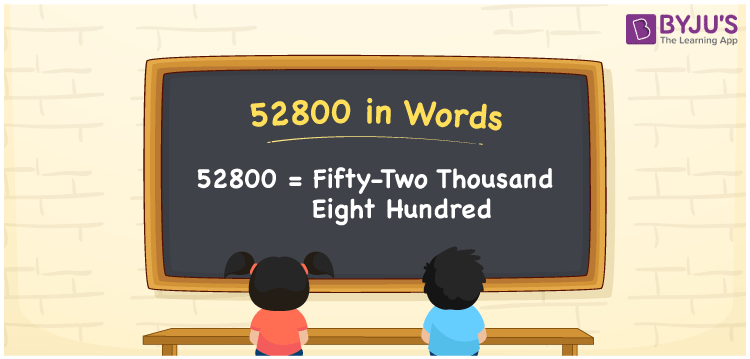# 52800 in Words

We can write 52800 in words as Fifty-two thousand eight hundred. If you spent Rs. 52800 for the renovation of a cabin, you can say, “I spent Fifty-two thousand eight hundred rupees to renovate the cabin”. In this article, you will learn how to write the cardinal number 52800 in word form using a place value chart and some facts about 52800.

 52800 in words Fifty-two thousand eight hundred Fifty-two thousand eight hundred in Numbers 52800

## 52800 in English words

We generally express numbers in words using the English alphabet. So, we spell 52800 in English as “Fifty-two thousand eight hundred”.## How to Write 52800 in Words?

The below table depicts the place value chart for the number 52800. Using this table, we can convert the number 52800 into words, as explained below.

 Ten thousands Thousands Hundreds Tens Ones 5 2 8 0 0

Here, ones = 0, tens = 0, hundreds = 8, thousands = 2, ten thousands = 5.

Let us expand these digits with the respective place values given above.

5 × Ten thousand + 2 × Thousand + 8 × Hundred + 0 × Ten + 0 × One

= 5 × 10000 + 2 × 1000 + 8 × 100 + 0 × 10 + 0 × 1

= 50000 + 2000 + 800

= Fifty thousand + Two thousand + Eight hundred

= Fifty-two thousand eight hundred

Thus, 52800 in words = Fifty-two thousand eight hundred.

52800 is a natural number that is the successor of 52799 and the predecessor of 52801.

52800 in words – Fifty-two thousand eight hundred

Is 52800 an odd number? – No

Is 52800 an even number? – Yes

Is 52800 a perfect square number? – No

Is 52800 a perfect cube number? – No

Is 52800 a prime number? – No

Is 52800 a composite number? – Yes

## Frequently Asked Questions on 52800 in Words

Q1

### Write the number 52800 in word form.

We can write the number 52800 in word form as Fifty-two thousand eight hundred.
Q2

### How to write Rs. 52800 in words on a cheque?

On a cheque, we generally write Rs. 52800 in words as Fifty-two thousand eight hundred rupees only.
Q3

### What is the difference between Fifty-two thousand eight hundred and Five thousand five hundred?

Fifty-two thousand eight hundred = 52800 Five thousand five hundred = 5500 Difference between Fifty-two thousand eight hundred and Five thousand five hundred = 52800 – 5500 = 47300